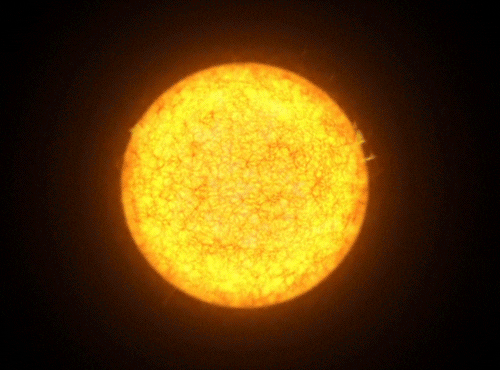# The (Alternative) Reason for the Seasons, Part 2: Variable Star

Part 1

On Earth, we have seasons due to our planet's tilt. In my last question, I proposed an alternative world where the seasons were instead caused by an eccentric orbit. Now I'm going for something more exotic. Instead of axial tilt or orbital eccentricity, what if the seasons were caused by actual variations in the energy output of the planet's host star?Details:

• The planet is earth-like and inhabited.
• The system must be stable for at least 3,000 years.
• The length of the "seasonal" cycle must be within [-50%, +100%] of one Earth year (i.e. between six and 24 months).
• The temperature difference for some point on the planet's surface between "winter" and "summer" must be at least 15oF and no more than 40oF.
• The cycle doesn't have to be exactly the same as Earth's, and all of the above can be taken loosely in light of that. For instance, the cycle could repeat once every four Earth years as long as there's a cold season of some description every one Earth year, and a 60oF temperature delta is acceptable so long as it happens less often than once per hot season.

# Is it possible for a real variable star system to produce seasons with these characteristics?

And if so, what kind of variable star would be best suited to producing such a world?

If not, how close can we get? Which variables are problematic?

Importantly, the planet does not need to have formed in its current location around the star, and the system does not have to be stable across astronomical timespans. I'm not concerned about whether an inhabitable planet could realistically form and evolve life in this scenario, just whether such a planet could have seasons caused by a variable star.

Let's look at the two extremes: $\Delta T=15^{\circ}\text{F}$ and $\Delta T=40^{\circ}\text{F}$. In the first case, I'll say $T_{\text{min}}=50^{\circ}\text{F}$ ($283.2\text{ K}$) and $T_{\text{max}}=65^{\circ}\text{F}$ ($291.5\text{ K}$). This gives us $$\frac{T_{\text{max}}}{T_{\text{min}}}=1.03$$ Let's also assume that the planet's surface temperature is well-approximated by its effective temperature; in other words, $$T\simeq T_{\text{eff}}=CL_*^{1/4}$$ where $C$ is a function that doesn't depend on $T$ or $L_*$. Let's say that $L_{*,\text{min}}$ and $L_{*,\text{max}}$ are the stellar luminosities that correspond to the minimum and maximum temperatures of the planet. Doing some substitution, we find that $$\frac{L_{*,\text{max}}}{L_{*,\text{min}}}=1.13$$ which is actually quite small for variable stars. Now, let's convert this to a change in absolute magnitude, $m$. We have $$\Delta m=-2.5\log_{10}\left(\frac{L_{*,\text{max}}}{L_{*,\text{min}}}\right)$$ which gives us $\Delta m=-.133$ magnitudes (remember, brighter objects have smaller magnitudes).

In the second case, say $T_{\text{min}}=35^{\circ}\text{F}$ ($274.8\text{ K}$) and $T_{\text{max}}=75^{\circ}\text{F}$ ($297.0\text{ K}$). This gives us $$\frac{T_{\text{max}}}{T_{\text{min}}}=1.08$$ We then have $$\frac{L_{*,\text{max}}}{L_{*,\text{min}}}=1.63$$ and so $\Delta m=-0.53$ magnitudes.

The best kind of variable stars I can find that meet your requirements are Alpha2 Canum Venaticorum variables, which have periods of up to roughly 160 days and magnitude changes of up to 0.1 magnitudes (possibly more). These stars should produce effects similar enough to what you want, within reasonable amounts.

It is true that this type of star - massive and luminous - will not last very long, and it will be difficult - not impossible, but difficult - for planets (and life!) to form around it. However, as the last paragraph of the question states,

Importantly, the planet does not need to have formed in its current location around the star, and the system does not have to be stable across astronomical timespans. I'm not concerned about whether an inhabitable planet could realistically form and evolve life in this scenario

If anyone can find a longer-lived star that has similar pulsations, that would fantastic. For now, though, I think this is the best you'll get.

• You are using T_min = 15F and T_max = 40F. But the OP wants the delta between T_min and T_max to be between 15 and 40F. Since your delta is 40-15=25F, you answered the question correctly, but you missed that when you read it. Mar 6, 2017 at 19:53
• @kingledion You're absolutely right. Thank you. I'll make an edit about that tomorrow to show some more numerical values based on that range (I also have some other information to add). Mar 7, 2017 at 1:35
• @kingledion I've fixed it. Mar 7, 2017 at 17:21

Variable stars that have a fixed repeating period do exist. They are known as cepheid variables and were important in astronomy as the period tells you the brightness of the star, and thus you can figure out the distance.

Look over the linked article: they are 4–20 times more massive than the Sun, and up to 100,000 times more luminous.

However, the periods only go up to about 9 months. The longer the period, the more luminous the star. I presume the luminosity varies with the mass (like normal stars), so we are looking at the largest stars, or 20 solar masses.

The other bad news is that such a star will only last for 5½ million years. So don’t expect planets to settle down and life to form in-place!

• Thank you for your answer! I feel, however, that an important point hasn't been addressed. Cepheids seem to undergo massive fluctuations, up to two magnitudes (that's a factor of 4 increase in brightness). That's only visible radiation presumably, but still, it seems to me that the change in the stars' energy output during a cycle would be too large for anything to survive both extremes. Oct 3, 2016 at 18:59
• The universe doesn't have what you’re looking for. The settlement needs to dig in and deal with the extremes, relying on the rock to buffer the temperature to the average. Oct 4, 2016 at 1:27